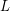# The circular embedding conjecture

 Importance: High ✭✭✭
 Author(s): Haggard, Gary
 Subject: Graph Theory » Basic Graph Theory » » Cycles
 Keywords: cover cycle
 Recomm. for undergrads: no
 Posted by: mdevos on: March 7th, 2007
Conjecture   Every 2-connected graph may be embedded in a surface so that the boundary of each face is a cycle.

This conjecture implies the cycle double cover conjecture, since the list of cycles which bound faces covers each edge exactly twice. Letbe a cubic graph, letbe a list of cycles covering every edge ofexactly two times, and form a topological space by gluing a disc to each circuit in. This space is a surface, and every face is bounded by a cycle. Thus, the circular embedding conjecture and the cycle double cover conjecture are equivalent for cubic graphs. For general graphs, this construction may fail since the neighborhood of a vertex may not be a disc (it could be a pinchpoint).

A stronger variant of this conjecture asserts that it is possible to find an embedding as above with the added condition that the dual graph is 5-colorable. This variant implies The five cycle double cover conjecture since the circuits bounding faces of a given color class may be grouped into a cycle. Next we state a different strengthening which asserts that we may find an embedding as above into an orientable surface.

Conjecture  (The oriented circular embedding conjecture)   Every 2-connected graph may be embedded in an orientable surface so that the boundary of each face is a circuit.

If this conjecture is true, then the oriented cycle double cover conjecture (see cycle double cover) is also true, since the list of circuits bounding faces all traversed in the clockwise direction cover each edge exactly once in each direction (since the surface is orientable, we may specify a global clockwise orientation). As was the case above, the oriented circular embedding conjecture is equivalent to the oriented cycle double cover conjecture for cubic graphs. Also as above, there is a strengthening of this conjecture which asserts that the graph may be embedded so that the dual graph is 5-colorable. If true, this would imply The orientable five cycle double cover conjecture. Novoline Spielautomaten online spielen. Das weltberühmte Book of Ra online spielen und Jackpot knacken!

## Bibliography

[H] G. Haggard, Edmonds characterization of disc embeddings. Proceedings of the Eighth Southeastern Conference on Combinatorics, Graph Theory and Computing (Louisiana State Univ., Baton Rouge, La., 1977), pp. 291--302. MathSciNet

* indicates original appearance(s) of problem.